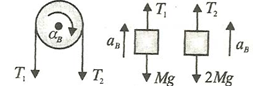# A cord is wrapped on a pulley (disk) of massand radiusas shown in Figure. To one end of the cord, a block of massis connected as shown and to other end in (a) a force of 2and in (b) a block of mass.Let angular acceleration of the disk inandisand, respectively, then (cord is not slipping on the pulley)a)b)c)d) None of these

## Question ID - 100197 :- A cord is wrapped on a pulley (disk) of massand radiusas shown in Figure. To one end of the cord, a block of massis connected as shown and to other end in (a) a force of 2and in (b) a block of mass.Let angular acceleration of the disk inandisand, respectively, then (cord is not slipping on the pulley)a)b)c)d) None of these

3537

(b)

In Fig,From Figure,So,Next Question :

Three ballsandof masses 2 kg, 4 kg and 8 kg, respectively, move along the same straight line and in the same direction, with velocities 4 m/s, 1 m/s and 3/4 m/s. Ifcollides withand subsequentlycollides with, find the velocity of balland ballafter collision, taking the coefficient of restitution as unitya)b)c)d)